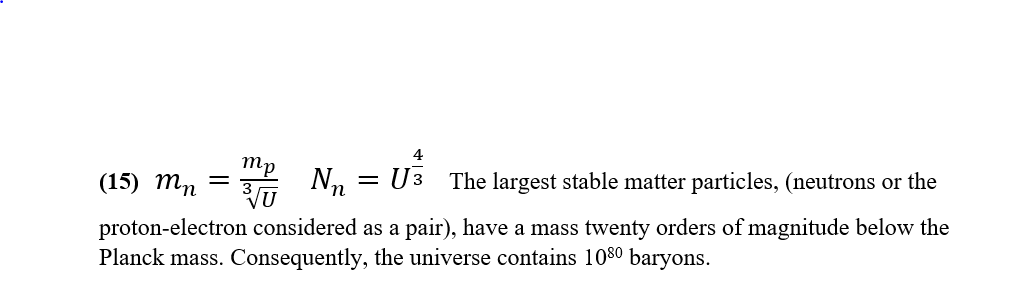## Hypersphere CosmologyAlternative to big-bang

Monday, 22 February 2021 16:56

## Equation 15Equation 15

It may seem odd that whilst the ‘enhanced’ Planck length and Planck time set a lower limit for quantum particles, (see equation 14) whilst a similarly ‘reduced’ Planck mass sets an upper limit for the size of stable quanta.

However, the wavelength of a quantum is inversely proportional to its mass, heavier quanta have smaller not larger wavelengths.

Stable quanta cannot have a mass in excess of e-20 Planck mass or their wavelengths would fall below the pixilation level of the universe.

See below: -

More in this category: « Equation 16 Equation 14 »
• #### HyperSphere Cosmology +Hypersphere Cosmology. (6)    P J Carroll 28/2/2021   Abstract.
• #### Type 1A supernovae and Hypersphere Cosmology +

Lensing, Redshift and Distance for Type1A Supernovae. All data input from Perlmutter et all, https://arxiv.org/pdf/astro-ph/9812133.pdf The measured Apparent Magnitudes of
• #### Galactic Rotation Curves +

Galactic Rotation Curves. A large discrepancy exists between the rotation curves for disc galaxies expected from a classical or relativistic
• #### Equation 17 +

Equation 17 The Redshift-Distance and the Lensing equations 6 and 16 together yield a derivation for the Antipode length L
• #### Equation 16 +

Equation 16 A stereographic projection can show the effect of projecting an n-sphere into an n-dimensional space. For
• #### Equation 15 +

Equation 15 It may seem odd that whilst the ‘enhanced’ Planck length and Planck time set a lower limit for
• #### Equation 14 +

Equation 14 The universe has an information deficit in the sense that it has only one bit of information/entropy for
• #### Equation 13 +

Equation 13 The universe has an information deficit in the sense that it has only one bit of information/entropy for
• #### Equation 12 +

Equation 12 The Bekenstein-Hawking conjecture arose to explain the behaviour of entropy in a black hole. https://en.wikipedia.org/wiki/Black_hole_thermodynamics#Overview It asserts that
• #### Equation 11 +

Equation 11 The Dirac Large Numbers Hypothesis suggests that a huge dimensionless number, or perhaps just a few huge dimensionless
• #### Equation 10 +

Equation 10 Here we show the modification to Newtonian Dynamics expected in a positively curved spacetime that has a negative
• #### Equation 9 +

Equation 9 Galaxies rotate but they do not rotate in accordance with our standard ideas about gravity. A large discrepancy
• 1
• 2